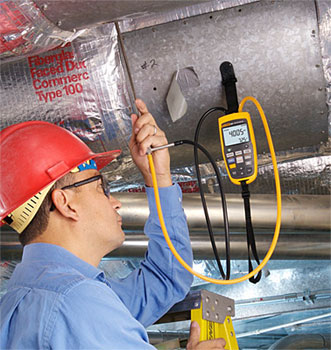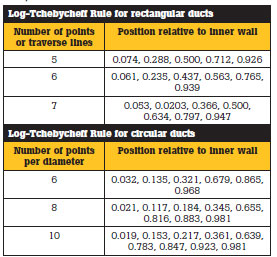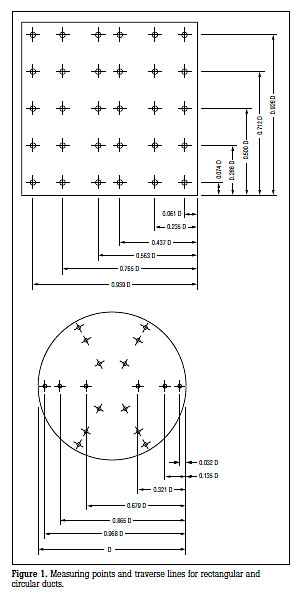# How to Make a Duct Traversal Airflow Measurement

Published with permission from Fluke®Why traverse
Proper air flow within HVAC ducts is essential to good equipment performance. When air flows are incorrect, the air can’t be conditioned as designed, operating costs are elevated, and equipment life expectancy is shortened.

Many circumstances warrant measuring air velocities or air flow, and a duct traverse is the most precise method of obtaining that information. A duct traverse consists of a number of regularly spaced air velocity and pressure measurements throughout a cross sectional area of straight duct (see Figure 1). This application note explains how to do that.

Conducting a duct traverse
For maximum airflow accuracy, take several readings across a traverse plane, convert them to velocity, and then average them. The illustration in Figure 1 shows the points along the traverse plane where you should take the measurements, in either rectangular or circular ducts.

Take airflow measurements at a minimum of 25 points, regardless of duct size.

• For duct sides shorter than 30”, five traversal points must be taken (5 on each side, 5*5=25).
• For duct sides of 30 through 36”, six points must be taken.
• For duct sides longer than 36”, seven points must be taken.
• If the duct side is less than 18”, then any readings you take should be taken from the center of equal areas that are no more than 6” apart, with a minimum of two points per side of the duct.

The preferred location of the traverse in a supply duct should be in a straight section of duct with 10 straight equivalent duct diameters upstream, and 3 straight equivalent duct diameters downstream of the traverse plane, although a minimum of 5 duct equivalent diameters upstream and 1 duct equivalent diameter downstream may give adequate results.

When the traverse is located close to the fan, flow conditions are usually more favorable upstream on the return side. The traverse on the upstream side should be 0.5 duct equivalent diameters upstream of the fan inlet.

Equivalent duct diameter = v (4HV/p)
H = horizontal duct dimension
V = vertical duct dimension
p = 3.14

Inserting the measuring device
To determine the insertion depth of the measuring device, reference the following tables. Let’s assume we have a sample duct with sides measuring 24” x 15”. For the 24” side of our sample rectangular duct, reference the “5 traverse lines” row.Note the five multipliers listed under “Position Relative to Inner Wall.” Multiply the duct dimension (24”) by the numbers in the table to arrive at the various insertion depths for that side of the duct. For example, the position closest to the inner wall would be: 0.074 * 24” = 1.78”, and so on. For the 15” side, follow the text instructions above for taking measurements when duct sides are less than 18”.

Step-by-Step
Here’s how to take the velocity and pressure measurements, using a Fluke 922.

• Connect the total pressure tube to the 922 “ ” port, and connect the static pressure tube to the “-” port.
• Select “Flow Volume” mode
• Select either round or rectangular duct
• Enter inside duct dimensions as prompted
• Zero meter
• Position the Pitot-Static tube tip within the duct at the first traverse point.
• When a stable air volume reading is displayed, press “Save” to store the reading.
• Repeat for each traverse point
• After all traverse point readings have been saved, press “Calc Average” for average airflow

Total pressure minus static pressure equals velocity pressure. The Fluke 922 converts velocity pressure to velocity automatically when in Velocity mode. In Flow Volume mode, the 922 will prompt for duct geometry and dimensions in order to display air flow (cfm) directly in real time. The 922 velocity and air flow calculations are based on standard air at 29.92” hg at 70° F.

Tips
When we talk about positioning the pitot tube 10 straight duct diameters upstream and 3 straight duct diameters downstream of the tranverse plane, we need to first convert rectangular duct measurements into their equivalent circular diameters.

To perform a traversal with circular duct, essentially follow the same traversal plane placement rules used with rectangular. However, circular ducts require measuring across 3 diameters (Figure 1), with a minimum of 6 measurements per diameter. Multiply the number of points you’ll measure with the figure in the second half of table 1 to determine the measuring position relative to the inner wall of the duct.

Notes:
1. When performing a duct traverse, always ensure the nose of the Pitot tube is parallel to the duct wall and facing the airflow.

2. Take readings in long, straight runs of duct, where possible. Avoid taking readings immediately downstream of elbows or other obstructions in the airway.